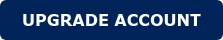Linearity in power loss models

Hello, everyone!

This is my first post here. I have a question regarding linearity in models of different power loss mechanisms, in both inductors and transformers.

On the one hand, I have seen many times a Fourier decomposition of wire losses, with an independent calculation at each harmonic frequency. The first step in the common approach is to determine the RMS values of the harmonics of the current intensity waveform. At each of these frequencies, the AC resistance is estimated with whichever model, and then the loss is calculated with the Joule heating equation: P = I²R. These powers are then all added up to obtain the total winding losses.

On the other hand, magnetic core losses models do not have such decomposition, at least none that I know of. I understand that there are fundamental non-linearities, mainly derived from the B-H loop nature. For example, the simple Steinmetz's equation (SE) is fine for a single sinusoidal waveform and its improved version, iGSE, may be used otherwise, but integrating the entire waveform, not using SE at each harmonic or something along those lines.

Considering linearity, I understand that superposition can be applied to the winding analysis. However, the basic skin depth equation or Dowell's method seem highly non-linear (with square roots, exponentials, and so on, all depending on frequency), so can anyone explain why is that approach correct with respect to the winding but not the core losses? As in if there are any elementary differences in their behavior or whether a similar process could also be carried out for core losses and it just hasn't been done.

Repliessays:

First, regarding the Wires-Loss, as you wrote correctly that for each harmonics you clculate ech winding resistanc by Dowell's method. Although this is a highly non-linear function of the aregument d/delta(f) (wire-diameter vs skin-depth), which is also highly non-linear in the freq. f, nevertheless, the calculated resistanse of each winding is not dependent by the winding's current magnitude. So, each of the windings has its own resistance per given a harmonic-freq. As you know the rms of each winding's-current the power per winding is as you wrote. The relationships between the curren a are described by simple algbra in the the Laplace domain, and they are Linear. Hence, you simply sum the power-loss of the windings. The resulat is just a simple real scalar - Ptot(Harmonic's freq.)! If overal level of exitation changes, than it's am linear change of currents/voltages. Now, according to Furrier all electrical variables of any Harmonic-Freq are Orthogonal to the same variable in a different Harmonic-freq. Hence, you can simply sum the powers of the harmonics. (That's why Furrier is so powerfull!). So far it was very simple, and I hope it ios also clear.

Now, with regards to the Core-Losses, this is a completely different "animal". Remeber, the Hysteresis is non-linear in both the freq - f and the core's Induction-Density: B=B{f,H[Ij(f)]}.So, now the flux response to changes in Exitation's Magnitude, will cause non-linear changes in the Currents {Ij}. Hence, even at the same freq - f this is a non-linear system! From Hysteresis stand point, what's happening here is exitation changes causing the magnetic-flux-density to move from one Minor-Loop to a diffrent one, which is either larger or smaller. Thus each Minor-Loop has a different Area <==> Power-Loss. These minor-Loops are non-linearly dependent in both Freq-f & B.

I hope that could convince you. I just also hope that Dr. Moline wouldn't find any floaw in my arguments.

Published: 1 month agosays:

This is a good question.  Dowell's method calculates resistance factors, but the R to be used increases with frequency.  It is possible to create linear R-L networks that closely match the ac resistances predicted by Dowell.  In other words, the ac resistances produced by the models are a nonlinear function of frequency, but the equivalent circuits themselves are linear functions of the current.  The cores, however, are truly nonlinear.

This paper has a very thorough explation of the topic.

J. P. Vandelac and P. D. Ziogas, "A novel approach for minimizing high-frequency transformer copper losses," in IEEE Transactions on Power Electronics, vol. 3, no. 3, pp. 266-277, July 1988

https://ieeexplore.ieee.org/document/17944

Published: 3 weeks ago
Edited byon 3 weeks beforesays:

Thanks a lot for your answer, Verimod! I've found that reference very useful

Published: 1 week ago